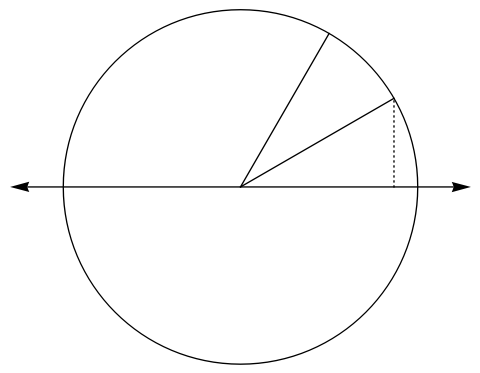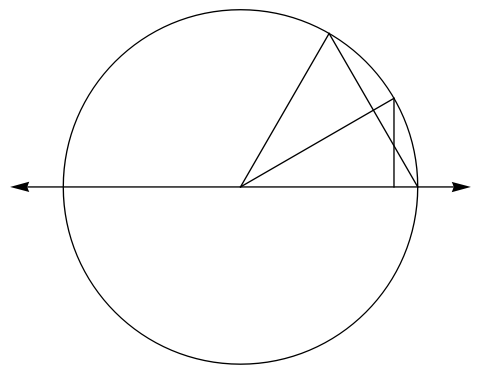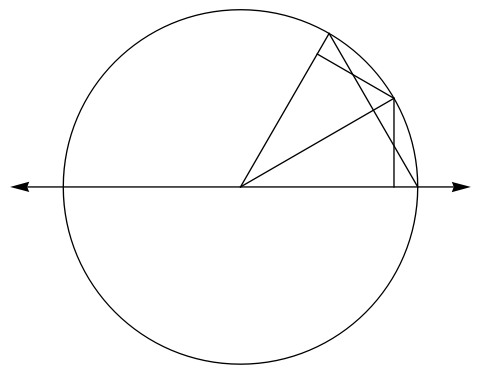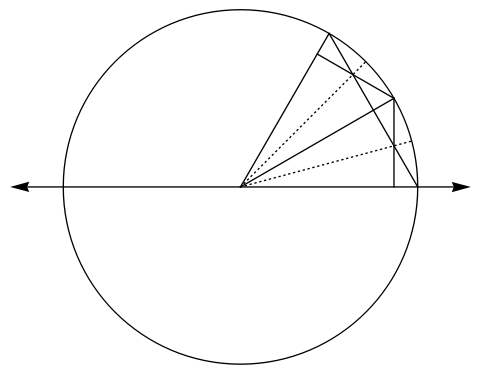# Leif's Website

## A geometric proof of $\sin 2\theta = 2 \sin \theta \cos \theta$

To start with, let's draw the standard trigonometric unit circle diagram for the angles $\theta$ and $2\theta$:The area corresponding to $\sin \theta \cos \theta$ is easy to see—it's the lower right-angle triangle. The area corresponding to $\sin 2 \theta$ is slightly harder to see. Since the height of the the $2\theta$ point is $\sin 2 \theta$ the triangle with base length $1$ will also have area $\frac{1}{2} \sin 2 \theta$. Thus the diagram becomes:The area of the big triangle is $\frac{1}{2} \sin 2 \theta$ and the area of the small triangle is $\frac{1}{2} \sin \theta \cos \theta$. We want to show that the area of the big triangle is twice the area of the small triangle. The symmetry of the diagram begs for another small triangle to be placed:The areas of the kite and the triangle are equal, and hence $\sin 2 \theta = 2 \sin \theta \cos \theta$. This is seen by symmetry along the dotted lines below: